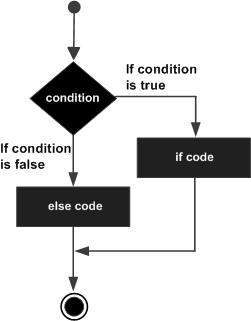# VB.Net - If...Then...Else Statement

Advertisements

An If statement can be followed by an optional Else statement, which executes when the Boolean expression is false.

## Syntax

The syntax of an If...Then... Else statement in VB.Net is as follows −

```If(boolean_expression)Then
'statement(s) will execute if the Boolean expression is true
Else
'statement(s) will execute if the Boolean expression is false
End If
```

If the Boolean expression evaluates to true, then the if block of code will be executed, otherwise else block of code will be executed.

## Flow Diagram## Example

```Module decisions
Sub Main()
'local variable definition '
Dim a As Integer = 100

' check the boolean condition using if statement
If (a < 20) Then
' if condition is true then print the following
Console.WriteLine("a is less than 20")
Else
' if condition is false then print the following
Console.WriteLine("a is not less than 20")
End If
Console.WriteLine("value of a is : {0}", a)
Console.ReadLine()
End Sub
End Module
```

When the above code is compiled and executed, it produces the following result −

```a is not less than 20
value of a is : 100
```

## The If...Else If...Else Statement

An If statement can be followed by an optional Else if...Else statement, which is very useful to test various conditions using single If...Else If statement.

When using If... Else If... Else statements, there are few points to keep in mind.

• An If can have zero or one Else's and it must come after an Else If's.

• An If can have zero to many Else If's and they must come before the Else.

• Once an Else if succeeds, none of the remaining Else If's or Else's will be tested.

## Syntax

The syntax of an if...else if...else statement in VB.Net is as follows −

```If(boolean_expression 1)Then
' Executes when the boolean expression 1 is true
ElseIf( boolean_expression 2)Then
' Executes when the boolean expression 2 is true
ElseIf( boolean_expression 3)Then
' Executes when the boolean expression 3 is true
Else
' executes when the none of the above condition is true
End If
```

## Example

```Module decisions
Sub Main()
'local variable definition '
Dim a As Integer = 100
' check the boolean condition '
If (a = 10) Then
' if condition is true then print the following '
Console.WriteLine("Value of a is 10") '
ElseIf (a = 20) Then
'if else if condition is true '
Console.WriteLine("Value of a is 20") '
ElseIf (a = 30) Then
'if else if condition is true
Console.WriteLine("Value of a is 30")
Else
'if none of the conditions is true
Console.WriteLine("None of the values is matching")
End If
Console.WriteLine("Exact value of a is: {0}", a)
Console.ReadLine()
End Sub
End Module
```

When the above code is compiled and executed, it produces the following result −

```None of the values is matching
Exact value of a is: 100
```
vb.net_decision_making.htm
Advertisements